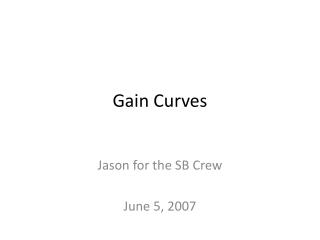DownloadDownload PresentationGain Curves

# Gain Curves

Télécharger la présentation## Gain Curves

- - - - - - - - - - - - - - - - - - - - - - - - - - - E N D - - - - - - - - - - - - - - - - - - - - - - - - - - -
##### Presentation Transcript

1. Gain Curves Jason for the SB Crew June 5, 2007

2. Raw 55Fe Spectra • Start with 55Fe spectrum from a MCA. • Fit peak to gaussian. • Calibration from a pulser turns channels into electrons. • Mean of gaussian corresponds to signal from 109 electrons (in CF4).

3. Raw Curves • Procedure: • 14 hour gain saturation curve • to fully charge up the stack • waited ~2 hours with voltage on • to verify stable gain • measured gain as a function of dV • source rate: ~100 Hz

4. Raw Curves • Procedure: • 14 hour gain saturation curve • to fully charge up the stack • waited ~2 hours with voltage on • to verify stable gain • measured gain as a function of dV • source rate: ~100 Hz

5. P/T Corrections • sealed volume in gain test box allows for easy P/T mapping. • in very good agreement with WIS. • Therefore, gain can be adjusted for P/T variations…

6. P/T Corrected Curves • Procedure: • 14 hour gain saturation curve • to fully charge up the stack • waited ~2 hours with voltage on • to verify stable gain • measured gain as a function of dV • corrected for P/T !! • source rate: ~100 Hz

7. P/T Corrected Curves • Procedure: • 14 hour gain saturation curve • to fully charge up the stack • waited ~2 hours with voltage on • to verify stable gain • measured gain as a function of dV • corrected for P/T !! • source rate: ~100 Hz

8. Gain Saturation Curve • Sample gain every two minutes for 14 hours. • Fit saturation curve to saturating exponential. • Gives the ability to extrapolate gain.

9. Completely Corrected Curves • Procedure: • 14 hour gain saturation curve • to fully charge up the stack • waited ~2 hours with voltage on • to verify stable gain • measured gain as a function of dV • corrected for P/T !! • use extrapolated saturation curve to correct for time… • SB sees a factor of ~2 • source rate: ~100 Hz

10. Completely Corrected Curves • Procedure: • 14 hour gain saturation curve • to fully charge up the stack • waited ~2 hours with voltage on • to verify stable gain • measured gain as a function of dV • corrected for P/T !! • use extrapolated saturation curve to correct for time… • SB sees a factor of ~2 • source rate: ~100 Hz

11. Completely Corrected Curves • Procedure: • 14 hour gain saturation curve • to fully charge up the stack • waited ~2 hours with voltage on • to verify stable gain • measured gain as a function of dV • corrected for P/T !! • use extrapolated saturation curve to correct for time… • source rate: ~100 Hz **note** the WIS data is from two different stacks… a gem was exchanged. --maybe not comparable.